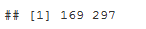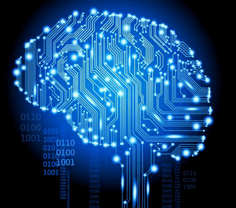# Time Series Analysis Using Max/Min… and some Neuroscience.

June 6, 2016
By

(This article was first published on R - Data Science Heroes Blog, and kindly contributed to R-bloggers)### IntroductionTime series have maximum and minimum points as general patterns. Sometimes the noise present on it causes problems to spot general behavior.

In this post, we will smooth time series -reducing noise- to maximize the story that data has to tell us. And then, an easy formula will be applied to find and plot max/min points thus characterize data.

### What we have

``````# reading data sources, 2 time series

# plotting...
plot(t1\$ts1, type = 'l')
plot(t2\$ts2, type = 'l')

``````As you can see there are many peaks, but intuitively you can imagine a more smoother line crossing in the middle of the points. This can achieved by applying a Seasonal Trend Decomposition (STL).

### Smoothing the series

``````# first create the time series object, with frequency = 50, and then apply the stl function.
stl_1=stl(ts(t1\$ts1, frequency=50), "periodic")
stl_2=stl(ts(t2\$ts2, frequency=50), "periodic")
``````

Important: If you don’t know the `frequency` beforehand, play a little bit with this parameter until you find a result in which you are comfortable.

More STL info

### Finding max and min

Creating the functions…

``````ts_max<-function(signal)
{
points_max=which(diff(sign(diff(signal)))==-2)+1
return(points_max)
}

ts_min<-function(signal)
{
points_min=which(diff(sign(diff(-signal)))==-2)+1
return(points_min)
}
``````

### Visualizing the results!

``````trend_1=as.numeric(stl_1\$time.series[,2])

max_1=ts_max(trend_1)
min_1=ts_min(trend_1)

## Plotting final results
plot(trend_1, type = 'l')
abline(v=max_1, col="red")
abline(v=min_1, col="blue")

``````With the line: `stl_1\$time.series[,2]` we are accessing the time series `trend` component. This is the smoothing method we will use, but there are others.

This first series has 3 maximums (red line) and 2 minimums (blue line) in the following places:

``````# When the max points occurs:
max_1
````````````# When the min points occurs:
min_1
``````### Comparing two time series

``````trend_2=as.numeric(stl_2\$time.series[,2])

max_2=ts_max(trend_2)
min_2=ts_min(trend_2)

# create two aligned plots
par(mfrow=c(2,1))

## Plotting series 1
plot(trend_1, type = 'l')
abline(v=max_1, col="red")
abline(v=min_1, col="blue")

## Plotting series 2
plot(trend_2, type = 'l')
abline(v=max_2, col="red")
abline(v=min_2, col="blue")

``````Some conclusions from both plots:

• `Series 2` starts with a `min` while 1 does with a `max`
• `Series 1` has 3 `max` and 2 `min`, just the opposite to the other series

Why is this important? Because of the nature of the data, which is in next section.

### What is this data about?`ts1` and `ts2` are two typical responses to a brain stimulus, in other words: what happens with the brain when a person looks at a picture / move a finger / think in a particular thing, etc… Electroencephalography.

Some studies in neuroscience focus on averaging several responses to one stimulus -for example, to look at one particular picture. They present several times a particular image to the person. Averaging all of these signal/time series, you get the typical response.

Then you can predict based on the similarity between this typical response and the new image (stimulus) that the person is looking at.

#### Typical response (or Event Related Potential)It’s important to get the when the positive peaks occur. In this case they are: `P1`, `P2` and `P3`. The same goes for the negative ones.

Note: It´s a common practice to invert negative and positive values.

#### Finally…

Typically the signal time length for this kind of studies last for 400ms, thus 1 point per millisecond, just the displayed plots. And the amplitude is in volts, (actually micro-volts). The same unit of measurement used by the notebook you are using now 😉###### that’s all!More DSH posts!

R-bloggers.com offers daily e-mail updates about R news and tutorials on topics such as: Data science, Big Data, R jobs, visualization (ggplot2, Boxplots, maps, animation), programming (RStudio, Sweave, LaTeX, SQL, Eclipse, git, hadoop, Web Scraping) statistics (regression, PCA, time series, trading) and more...